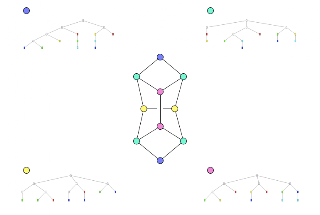Skip to main content

### Chemicals as colored graphs

The interface between maths and chemistry can be tricky when it comes to terminology - sets (maths) have elements, chemistry has a different kind of element; graphs have colors which are usually just numbers, diagrams of chemicals have colors which usually relate to the element type of the atom, and so on.

So, for maximum confusion, here are two pictures of graphs (that could represent chemical connectivity) colored by equivalence class (determined by signature). The signature trees are also drawn with graphical colors, but these represent the integer colors in the signature, which are not the same as the colors used to indicate equivalence class. Firstly, a structure that the smiles algorithm is meant to have trouble with (but may not exist):It looks quite strained, so I expect that it may not be possible to synthesise. Another multi-ring system is this one:I don't even know what this one would be called, even if it did exist. Annoyingly, this structure triggers a bug if the two dark blue atoms are connected. This makes the graph 3-regular, but the yellow equivalence class is split, which shouldn't happen.

### CommentsThis comment has been removed by the author.Gilleain, in the first graph, I do not see the equivalence of all four cyan nodes... the top two are not really equivalent to the bottom two, or are they? If so, why? To me, they seem to have different environments...gilleain said…
Ah top marks for spatial awareness, but only half for colour comparison :)

The upper two are what Rasmol used to call "Sea green", while the lower two are cyan. The trees on the right (which are the signatures) are arranged in the same layer order as the graph.Oh, wow... those are two different colors! Hahaha... :)

R has nice methods to create a list of colors where you pick the number of colors and it optimizes for contrast :)

### How many isomers of C4H11N are there?

One of the most popular queries that lands people at this blog is about the isomers of C4H11N - which I suspect may be some kind of organic chemistry question on student homework. In any case, this post will describe how to find all members of a small space like this by hand rather than using software.

Firstly, lets connect all the hydrogens to the heavy atoms (C and N, in this case). For example:

Now eleven hydrogens can be distributed among these five heavy atoms in various ways. In fact this is the problem of partitioning a number into a list of other numbers which I've talked about before. These partitions and (possible) fragment lists are shown here:

One thing to notice is that all partitions have to have 5 parts - even if one of those parts is 0. That's not strictly a partition anymore, but never mind. The other important point is that some of the partitions lead to multiple fragment lists - [3, 3, 2, 2, 1] could have a CH+NH2 or an NH+CH2.

The final step is to connect u…

### The Gale-Ryser Theorem

This is a small aside. While reading a paper by Grüner, Laue, and Meringer on generation by homomorphism they mentioned the Gale-Ryser (GR) theorem. As it turns out, this is a nice small theorem closely related to the better known Erdős-Gallai (EG).

So, GR says that given two partitions of an integer (p and q) there exists a (0, 1) matrixA iff p*dominatesq such that the row sum vector r(A) = p and the column sum vector c(A) = q.

As with most mathematics, that's quite terse and full of terminology like 'dominates' : but it's relatively simple. Here is an example:

The partitions p and q are at the top left, they both sum to 10. Next, p is transposed to get p* = [5, 4, 1] and this is compared to q at the bottom left. Since the sum at each point in the sequence is greater (or equal) for p* than q, the former dominates. One possible matrix is at the top left with the row sum vector to the right, and the column sum vector below.

Finally, the matrix can be interpreted as a bi…

### Havel-Hakimi Algorithm for Generating Graphs from Degree Sequences

A degree sequence is an ordered list of degrees for the vertices of a graph. For example, here are some graphs and their degree sequences:

Clearly, each graph has only one degree sequence, but the reverse is not true - one degree sequence can correspond to many graphs. Finally, an ordered sequence of numbers (d1 >= d2 >= ... >= dn > 0) may not be the degree sequence of a graph - in other words, it is not graphical.

The Havel-Hakimi (HH) theorem gives us a way to test a degree sequence to see if it is graphical or not. As a side-effect, a graph is produced that realises the sequence. Note that it only produces one graph, not all of them. It proceeds by attaching the first vertex of highest degree to the next set of high-degree vertices. If there are none left to attach to, it has either used up all the sequence to produce a graph, or the sequence was not graphical.

The image above shows the HH algorithm at work on the sequence [3, 3, 2, 2, 1, 1]. Unfortunately, this produce…